# Infinite version of the Set card game

edit 2023/06/17: I elaborated a bit more in the conclusion about the open problem of finding a minimal $\kappa$.

## The Set Game

I visited A Coruña last week for the Web Engines Hackfest and to participate to internal events with my fellow Igalians. One of our tradition being to play board games, my colleague Ioanna presented a card game called Set. To be honest I was not very good at it, but it made me think of a potential generalization for infinite sets that is worth a blog post…

Basically, we have a deck of $\lambda^\mu$ cards with $\mu = 4$ features (number of shapes, shape, shading and color), each of them taking $\lambda = 3$ possible values (e.g. red, green or purple for the color). Given $\kappa$ cards on the table, players must extract $\lambda$ cards forming what is called a Set, which is defined as follows: for each of the $\mu$ features, either the cards use the same value or they use pairwise distinct values.

Formally, this can be generalized for any cardinal $\lambda$ as follows:

• A card is a function from $\mu$ (the features) to $\lambda$ (the values).
• A set of cards $S$ is a Set iff for any feature $\alpha < \mu$, the mapping $\Phi_\alpha^S : S \rightarrow \lambda$

that maps a card $c$ to the value $c\left(\alpha\right)$ is either constant or one-to-one.

Given a value $\kappa$ such that $\lambda \leq \kappa \leq \lambda^\mu$, can we always extract a Set when $\kappa$ cards are put on the table? Or said otherwise, is there a set of $\kappa$ cards from which we cannot extract any Set?

## Trivial cases ($\lambda \leq 2$ or $\mu \leq 1$)

Given $\kappa \geq \lambda$ cards, we can always extract a Set $S$ in the following trivial cases:

• If $\mu = 0$ then the deck contains only one card $c = \emptyset$. If $\lambda \geq 2$ such a set of $\kappa$ cards does not exist. Otherwise we can just take $S = \emptyset$ or $S =\left\{\\left\{c\\right\}\right\}$: these are Sets since the definition is trivial for $\mu = 0$.
• If $\mu \geq 1$ and $\lambda = 0$ then the deck is empty. We take $S = \emptyset$ and for any $\alpha < \mu$, $\Phi_\alpha^\emptyset = \emptyset$ is both constant and one-to-one.
• If $\mu = 1$ and $\lambda \geq 1$, a card $c$ is fully determined by its value $c\left(0\right)$, so distinct cards give distinct values. So we can pick any $S$ of size $\lambda$: it is a Set since $\Phi_0$ is one-to-one.
• If $\lambda = 1$ then we can pick any singleton $S$: it is a Set since for any feature $\alpha < \mu$ the mapping $\Phi_\alpha^S$ is both constant and one-to-one.
• If $\lambda = 2$ then we can pick any pair of cards $S$: it is a Set since for any feature $\alpha < \mu$ the mapping $\Phi_\alpha^S$ is either constant or one-to-one (depending on whether the two cards display the same value or not).

👉🏼 For the rest of this blog post, I’ll assume $\mu \geq 2$ and $\lambda \geq 3$.

## Not enough cards on the table ($\kappa \leq \mu$)

If $\mu \geq \kappa \geq \lambda \geq 3$ then we consider cards $c_\alpha$ for each $\alpha < \kappa$ defined for each $\beta < \mu$ as $c_\alpha\left\{\left(\beta\right)\right\} = \delta_\left\{\alpha, \beta\right\}$ (using Kronecker delta). If we extract a subset $S$ from these cards and $\alpha_1, \alpha_2, \alpha_3 < \kappa \leq \mu$ are indices for elements of $S$ then $\Phi_\left\{\alpha_1\right\}^S$ respectively evaluates to 1, 0 and 0 for $\alpha_1, \alpha_2, \alpha_3$ so $S$ is not a Set.

👉🏼 For the rest of this blog post, we’ll assume $\mu < \kappa$ and will even focus on the minimal case $\kappa = \lambda$.

## Finite number of values ($\lambda < \aleph_0$)

Let’s consider a finite number of values $\lambda \geq 3$ and define the card $c_\alpha$ for each $\alpha < \lambda$ as follows: $c_\alpha\left(\beta\right) = \delta_\left\{\alpha, 0\right\}$ for $\beta=0$ (again Kronecker delta) and $c_\alpha\left(\beta\right) = \alpha$ for $0 < \beta < \mu$. Since $\mu \geq 2$, the latter case shows that $S = \\left\{ c_\alpha : \alpha < \lambda \\right\}$ contains exactly $\lambda$ cards. Since $\kappa = \lambda < \aleph_0$, the only way to extract a subset of size $\lambda$ would be to take all the cards. But they don’t form a Set since by construction $\left\{\Phi_0\left\{\left(c_0\right)\right\}\right\} = 1 \neq 0 = \left\{\Phi_0\left\{\left(c_1\right)\right\}\right\} = \left\{\Phi_0\left\{\left(c_2\right)\right\}\right\}$.

👉🏼 For the rest of the blog post, I’ll assume $\lambda$ is infinite.

## Singular number of values ($\mi\left\{cf\right\}\left(\lambda\right) < \lambda$)

If $\lambda$ is a singular cardinal, then we consider a cofinal sequence $\left\{\\left\{ \alpha_\left\{\gamma\right\}, \gamma < \nu \\right\}\right\} \subseteq \lambda$ of length $\nu < \lambda$ and define the card $c_\alpha$ for $\alpha < \lambda$ as follows:

• For $\beta = 0$, we consider the smallest ordinal $\gamma < \nu \leq \lambda$ such that $\alpha < \alpha_\gamma$ and define $c_\alpha\left\{\left(0\right)\right\} = \gamma$.
• For any $1 \leq \beta < \mu$, $\left\{c_\alpha\left\{\left(\beta\right)\right\}\right\} = \alpha$.

Since $\mu \geq 2$, the latter case shows that these are $\lambda$ distinct cards. Consider $S \subseteq \left\{\\left\{c_\alpha, \alpha < \lambda \\right\}\right\}$. If $\Phi_0^S$ evaluates to a constant value $\gamma < \nu$ then $S = \left\{\left\left(\Phi_0^S\right\right)\right\}^\left\{-1\right\}\left(\\left\{\gamma\\right\}\right)$ has size at most $\left\{|\alpha_\gamma|\right\} < \lambda$. If instead $\Phi_0^S$ is one-to-one then it takes at most $\nu$ distinct values so again $\left\{|S|\right\} \leq \nu < \lambda$. Hence $S$ is not a Set.

👉🏼 For the rest of the blog post, I’ll assume $\lambda$ is an infinite regular cardinal.

## Finite number of features ($\mu < \aleph_0$)

In this section, we assume that the number of features $\mu$ is finite. Let’s consider $\lambda$ cards $c_\alpha$ and extract a Set $S$ by induction as follows:

• $S_0 = \\left\{ c_\alpha : \alpha < \lambda \\right\}$
• For any $\beta < \mu$, we construct $S_\left\{\beta+1\right\} \subseteq S_\left\{\beta\right\}$ of cardinality $\lambda$. We note that $\lambda$ is regular and $S_\beta = \left\{\bigcup_\left\{\alpha \in \Phi_\beta^\left\{S_\beta\right\}\left\{\left(S_\beta\right)\right\}\right\} \left\{\left\left(\Phi_\beta^\left\{S_\beta\right\}\right\right)\right\}^\left\{-1\right\}\left\{\left(\\left\{\alpha\\right\}\right)\right\} \right\}$

so there are only two possible cases:

• If $\Phi_\beta^\left\{S_\beta\right\}\left\{\left(S_\beta\right)\right\}$ is of cardinality $\lambda$ then pick $\lambda$ elements from $S_\beta$ with pairwise distinct image by $\Phi_\beta^\left\{S_\beta\right\}$.
• Otherwise, if there is $\alpha < \lambda$ such that $\left\{\left\left(\Phi_\beta^\left\{S_\beta\right\}\right\right)\right\}^\left\{-1\right\}\left\{\left(\\left\{\alpha\\right\}\right)\right\}$ is of cardinality $\lambda$, then let it be our $S_\left\{\beta+1\right\}$.
• $S = S_\left\{\mu\right\}$

Then by construction, $S$ is of size $\lambda$ and for any $\beta < \mu$, $S \subseteq S_\left\{\beta+1\right\}$ which means that $\Phi_\beta^S = \left\{ \left\{\left(\Phi_\beta^\left\{S_\beta\right\}\right)\right\}_\left\{| S\right\}\right\}$ is either constant or one-to-one.

Incidentally, although I said I would focus on the case $\kappa = \lambda$ the result of this session shows that we can extract a Set if more than $\lambda$ cards are put on the table!

## Summary and open questions

Above are the results I found from a preliminary investigation, which can be summarized as follows:

1. If $\lambda \leq 2$ or $\mu \leq 1$ then we can always find a Set from $\kappa \geq \lambda$ cards.
2. If $3 \leq \lambda \leq \mu$ then for any $\kappa$ such that $\lambda \leq \kappa \leq \mu$ there is a set of $\kappa$ cards from which we cannot extract any Set.
3. If $2 \leq \mu < \lambda < \aleph_0$ there is a set of $\lambda$ cards from which we cannot extract any Set.
4. If $2 \leq \mu$ and $\lambda$ is singular then there is a set of $\lambda$ cards from which we cannot extract any Set.
5. If $2 \leq \mu < \aleph_0 \leq \left\{\mi\left\{cf\right\}\left(\lambda\right)\right\} = \lambda$, then we can always find a Set from $\kappa \geq \lambda$ cards.

Note that for the standard game $3 = \lambda < \mu = 4$ the only of the results above that applies is (2). Indeed, having only three or four cards on the table is generally not enough to extract a Set!

So far, I was not able to find an answer for the case $\aleph_0 \leq \mu < \left\{\mi\left\{cf\right\}\left(\lambda\right)\right\} = \lambda \leq \kappa$. It looks like the inductive construction from the previous paragraph could work, but it’s not clear what guarantees that taking intersection at limit step would preserve size $\kappa$ (an idea would be to use closed unbounded $S_\beta$ instead but I didn’t find a satisfying proof). I also failed to build a counter-example set of $\lambda$ cards without any Set subset, despite several attempts.

More generally, an open problem is to determine the minimal number of cards $\kappa$ (with $\lambda \leq \kappa \leq \lambda^\mu$) to put on the table to ensure players can always extract a Set subset… or even if such a number actually exists! If it does, then in cases (2) (3) (4) we only know $\kappa > \lambda$. In cases (1) and (5) the minimum value $\kappa = \lambda$ works ; and when $\mu \geq 2$ and $\lambda \geq 3$ are finite, the maximum value $\kappa = \lambda^\mu$ means taking the full deck, which works too (e.g. it always contains the Set given by $\forall \alpha < \lambda, \forall \beta < \mu, c_\alpha\left(\beta\right) = \alpha$). Incidentally, note that the latter case is consistent with (2) and (3) since we have $\lambda^\mu > \mu, \lambda$. But in general for infinite parameters putting $\kappa = \lambda^\mu$ cards on the table does not mean putting the full deck, so it’s less obvious whether we can extract a Set

# MathML in Chrome 109

2023/06/15: Most of this article was written in January 2023, but I only finished and published it in June. Hopefully, information is still up-to-date 😃

## TL;DR

Igalia announced early this year that MathML is back to Chromium. This is an excellent news, for those like me who read and write mathematics on the web. Native support for such a universal human language sounds uncontroversial but it has taken a quarter century to get MathML implemented in all web engines… I invite you to listen to the corresponding episode of Igalia Chats where you can find some rationale about why it took so long. In this blog post, I’ll try to elaborate a bit about technical challenges we were able to overcome, thanks to the introduction of MathML Core. To be honest, things are still not perfect, but at least we can now rely on a clean foundation and continue to iterate improvements and fix bugs!

## Timeline

As a reference, I’m providing below a list of important events (based on this timeline and this slide) for native MathML support in browsers:

## A simple fraction

One of the first thing you can notice is that a significant portion of the work was done by volunteer contributors at the time when the set of requirements to ship a feature or integrate it in the specification was very small. Let’s consider some basic MathML formula to illustrate that:

<math style="font: 32pt STIX Two Math;">
<mfrac>
<mspace width="2em" height="1em" depth=".5em" style="background: red"/>
<mspace width="2em" height=".5em" depth="1em" style="background: blue"/>
</mfrac>
[/itex]


The [itex] element is the root container for a new MathML formula. The <mfrac> element describes a fraction with two <mspace/> children corresponding respectively to its numerator and denominator. These <mspace/> elements are boxes of specified width, ascent and descent, as described by their width, height and depth attributes (using TEX terminology). Finally, the style attribute attaches inline style to MathML elements.

MathML 3 describes how to interpret the attributes of the <mspace> element. In general, it does not really define interaction with other web technologies and the behavior of the style attribute “is not specified”. However, browser implementers can safely assume it has the same syntax and semantic as HTML and that’s basically what MathML Core says. So we know the [itex] element uses the font family STIX Two Math and font size of 32pt, while the <mspace> elements have red and blue background.

But how should we layout the <mfrac> element?

## TEX-based layout

MathML 3 does not really say much more than the semantic “used for fractions” and the syntax <mfrac> numerator denominator </mfrac>. For the fraction bar, “the exact thickness of these is left up to the rendering agent”. MathML Core has a more detailed description involving vertical position and thickness of the fraction bar, minimal gaps between numerator/denominator and fraction bar as well as minimal shifts of the numerator/denominator’s baseline with respect to the baseline, horizontal centering of the numerator/denominator and more.

One of the essential part is that many of these parameters are taken from STIX Two Math’s Open Type MATH table such as fractionNumeratorShiftUp, fractionDenominatorGapMin etc and are generalizing the rules from the TEXBook. You may notice parameters like fractionNumeratorDisplayStyleShiftUp, fractionDenomDisplayStyleGapMin etc which are variants based on the math-style we will explain below.

In any case, as long as they follow the layout algorithm described in MathML Core, all browsers should now render the fraction example the same, right?

## Exposing MathML magic

Assuming STIX Two Math is available or provided as a web font, Chrome and Firefox renders the previous example that way:You can notice that the size of the numerator/denominator is different from what you get in Safari. Indeed, the web inspector shows that the <mspace> elements has a smaller font-size than the one of the [itex] or <mfrac> elements. This magic is suggested by the remaining part of the description of MathML 3 we have ignored so far:

The mfrac element sets displaystyle to “false”, or if it was already false increments scriptlevel by 1, within numerator and denominator.

Let’s try to explain this a bit more. The displaystyle and scriptlevel properties are concepts from MathML 3 which are themselves generalizing TEX. Imagine many nested superscripts: the font size is scaled down each time you enter a superscript, and becomes smaller and smaller. Here, the scriptlevel corresponds to the nesting level and it affects the font size. Regarding displaystyle=false, this is typically used when you want to render the formula with more compact height e.g. for formulas rendered inline within a paragraph of text.

So the MathML 3 spec essentially says that the subformulas numerator/denominator should be rendered with compact height, and if we are already in such a mode we should even scale down the font size within these subformulas. The only problem is that this behavior is not specified at all by CSS!

In order to solve that, we introduced the math-style and math-depth CSS properties, explaining how they affect font-size in the cascade. That way the behavior can be completely handled by the CSS engines. Then MathML Core describes how displaystyle/scriptlevel attributes are treated as presentational hints together with appropriate rules in the User Agent stylesheet. At the end everything is well-specified and Safari’s behavior is a bug.

## Integration with the web platform

The previous paragraphs described how we integrated TEX-based math layout inside the web platform. MathML 3 already started that by defined a SGML tree and HTML5 / MathML Core went further by defining the parsing rules, a WebIDL or integration with HTML and SVG.

The integration problem can also be seen on the other side: how do we interpret CSS features for math layout? For example what happens if one specifies a CSS width on the <mfrac> element? Or a padding? What would be the CSS layout if there are less or more children than just the numerator/denominator? What happen if float: left or position: absolute are specified on a child? Probably math authors don’t care too much but that’s still something that needs to be defined in the spec and implemented in a cross-compatible way.

Answering these questions were also useful for many reasons. Just to list a few examples off the top of my head:

• In order to make things manageable, MathML Core focuses on a susbet of MathML 3 and some people are interested in writing polyfills for legacy or future features, which is something that can often be done by CSS.
• Using a terminology that takes into account direction/writing-mode facilitates the implementation of right-to-left math and could allow vertical math in the future.
• To avoid visual confusion between the fraction bar and another adjacent items (e.g. minus sign or another fraction’s bar), a default 1-pixel space is added around the element. This can be easily done by setting the padding in the User Agent stylesheet… as long as it’s supported!
• Bypassing MathML layout in invalid subtrees (e.g. <mfrac> with three children) as was done in old implementations are making things harder for fuzzers and testcase reducers. Additionally these fuzzers often find issues happening with exotic CSS features, so better if the spec says how to handle them.

## Web Platform tests

We’ve seen some examples on how to improve the MathML specification. This leads to the topic of Web Platform tests, which are very important to verify conformance with that specification and ensure browser interoperability. That’s a good tool and motivation for implementers and they may also prevent regressions since they are run regularly, at least each time a change is made in the browser repository.

However, MathML 3 was using manual tests where one has to visually compare the rendering of the 1675 testcases in a browser against a sample rendering and decide whether it passes or not. That’s a quite tedious and not very robust way of testing!

Fortunately, this has changed with MathML Core with around 3000 tests following the format of Web Platform tests! Trying to analyze that a bit, Chrome has an excellent pass rate except for things that need to be clarified in the spec. Firefox and Safari are also doing good job but failing on tests for new behavior or features that got clarified by MathML Core.

A complementary metrics for interoperability is MDN’s browser-compat-data. You can find these compatibility data at the bottom of MDN pages, for example for the MathML article. Again, a quick analysis confirms that browsers are quite interoperable for MathML Core, possibly mixed with other web technologies, but not for new CSS features (implemented in Chromium) or legacy MathML features (remaining in Firefox and Safari).

## Accessibility

Abraham Nemeth who developed Braille code for mathematical notations, presented his view on math accessibility in the book Braille into the next millenium (2000):

The Principle of Meaning Versus Notation: In my view, it is the transcriber’s function to supply only notation, not meaning in an accessible form (speech or braille). It is the reader’s function to extract the meaning from the notation the transcriber supplies. Consider the common notation (x,y). That notation can mean many things: the ordered pair whose first component is x and second component is y ; the point in the cartesian coordinate with abscissa x and ordinate y; the open interval on the real line with left endpoint x and right endpoint y; or the greatest common divisor of x and y. The transcriber’s function, however, is only to convey this five-symbol expression to the reader. It is the reader’s function to extract whatever meaning his experience and the context of the text permit.

However, for people with different need, or for various uses cases, it is often important to provide more semantic. This is something various members from the MathML WG have worked on and many discussions are currently happening.

In any case, for native MathML implementation in browsers we are limited to what was called “presentation MathML” in legacy specifications, which gives a very small amount of information: an <mfrac> can be fraction, binomial coefficient etc ; an <msup> can be used to denote a power, a derivative, an index etc ; an <mtable> can be used for matrices, to perform table layout etc

As explained above, some effort was done to implement native accessibility support for MathML in WebKit/Gecko for various assistive technologies in Linux, macOS or Windows. For the present project, we didn’t try to do more than what currently exists. We only documented existing support in the MathML AAM specification and implemented it in Chromium. This is the bare minimum we can do to ensure the reader can follow Nemeth’s approach.

## Conclusion

As the main person who has led this effort, this is great personal achievement and I’d like to thank all the people who supported it, including my colleagues at Igalia, members of the MathML community, standardization groups, browser developers, web developers, sponsors and contributors to the Open Collective. Finally we made it! 🎉

# Update on OpenType MATH fonts

I mentioned in a previous post that Igalia organized the Web Engines Hackfest 2022 last week. As usual, fonts were one of the topic discussed. Dominik Röttsches presented COLRv1 color vector fonts in Chrome and OSS (transcript) and we also settled a breakout session on Tuesday morning. Because one issue raised was the availability of OpenType MATH fonts on operating systems, I believe it’s worth giving an update on the latest status…

There are only a few fonts with an OpenType MATH table. Such fonts can be used for math layout e.g. modern TeX engines to render LaTeX, Microsoft Office to render OMML or Web engines to render MathML. Three of such fonts are interesting to consider, so I’m providing a quick overview together with screenshots generated by XeTeX from the LaTeX formula $${\sqrt{\sum_{n=1}^\infty {\frac{10}{n^4}}}} = {\int_0^\infty \frac{2x dx}{e^x-1}} = \frac{\pi^2}{3} \in {\mathbb R}$$:

• Cambria Math is a proprietary font installed by default on Microsoft Windows.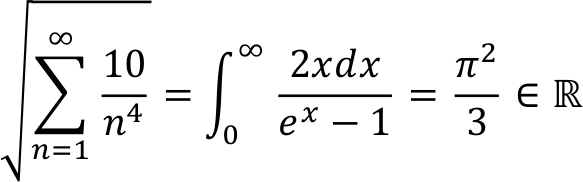• STIX Two Math is a font under the OFL license with a large unicode coverage for scientific characters.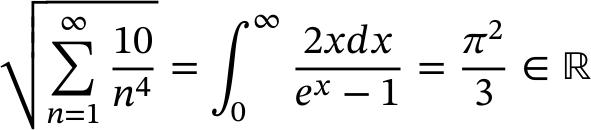• Latin Modern Math is a font under the GUST license with the Computer Modern style that TeX uses by default.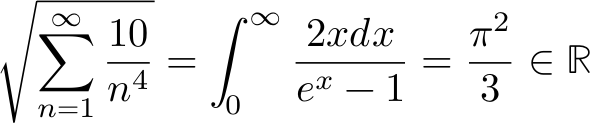Recently, Igalia has been in touch with Myles C. Maxfield who has helped with internal discussion at Apple regarding inclusion of STIX Two Math in the list of fonts on macOS. Last week he came back to us announcing it’s now the case on all the betas of macOS 13 Ventura 🎉 ! I just tested it this morning and indeed STIX Two Math is now showing up as expected in the Font Book. Here is the rendering of the last slide of my hackfest presentation in Safari 16.0: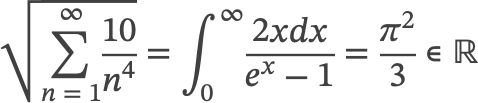Obviously, this is a good news for Chromium and Firefox too. For the former, we are preparing our MathML intent-to-ship and having decent rendering on macOS by default is important. As for the latter, we could in the future finally get rid of hardcoded tables to support the deprecated STIXGeneral set.

Another interesting platform to consider for Chromium is Android. Last week, there has been new activity on the Noto fonts bug and answers seem more encouraging now… So let’s hope we can get a proper math font on Android soon!

Finally, I’m not exactly sure about the situation on Linux and it may be different for the various distributions. STIX and Latin Modern should generally be available as system packages that can be easily installed. It would be nicer if they were pre-installed by default though…

# Short blog post from Madrid's hotel room

This week, I finally went back to A Coruña for the Web Engines Hackfest and internal company meetings. These were my first on-site events since the COVID-19 pandemic. After two years of non-super-exciting virtual conferences I was so glad to finally be able to meet with colleagues and other people from the Web.

Igalia has grown considerably and I finally get to know many new hires in person. Obviously, some people were still not able to travel despite the effort we put to settle strong sanitary measures. Nevertheless, our infrastructure has also improved a lot and we were able to provide remote communication during these events, in order to give people a chance to attend and participate !

Work on the Madrid–Galicia high-speed rail line finally completed last December, meaning one can now travel with fast trains between Paris - Barcelona - Madrid - A Coruña. This takes about one day and a half though and, because I’m voting for the Legislative elections in France, I had to shorten a bit my stay and miss nice social activities 😥… That’s a pity, but I’m looking forward to participating more next time!

Finally on the technical side, my main contribution was to present our upcoming plan to ship MathML in Chromium. The summary is that we are happy with this first implementation and will send the intent-to-ship next week. There are minor issues to address, but the consensus from the conversations we had with other attendees (including folks from Google and Mozilla) is that they should not be a blocker and can be refined depending on the feedback from API owners. So let’s do it and see what happens…

There is definitely a lot more to write and nice pictures to share, but it’s starting to be late here and I have a train back to Paris tomorrow. 😉

# Igalia's contribution to the Mozilla project and Open Prioritization

As many web platform developer and Firefox users, I believe Mozilla’s mission is instrumental for a better Internet. In a recent Igalia’s chat about the Web Ecosystem Health, participants made the usual observation regarding this important role played by Mozilla on the one hand and the limited development resources and small Firefox’s usage share on the other hand. In this blog post, I’d like to explain an experimental idea we are launching at Igalia to try and make browser development better match the interest of the web developer and user community.

## Igalia’s contribution to browser repositories

As mentioned in the past in this blog, Igalia has contributed to different part of Firefox such as multimedia (e.g. <video> support), layout (e.g. Stylo, WebRender, CSS, MathML), scripts (e.g. BigInt, WebAssembly) or accessibility (e.g. ARIA). But is it enough?

Although commit count is an imperfect metric it is also one of the easiest to obtain. Let’s take a look at how Igalia’s commits repositories of the Chromium (chromium, v8), Mozilla (mozilla-central, servo, servo-web-render) and WebKit projects were distributed last year: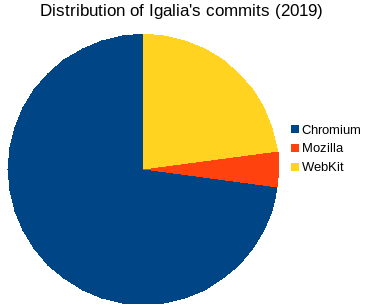Diagram showing, the distribution of Igalia's contributions to browser repositories in 2019 (~5200 commits). Chromium (~73%), Mozilla (~4%) and WebKit (~23%).

As you can see, in absolute value Igalia contributed roughly 3/4 to Chromium, 1/4 to WebKit, with a small remaining amount to Mozilla. This is not surprising since Igalia is a consulting company and our work depends on the importance of browsers in the market where Chromium dominates and WebKit is also quite good for iOS devices and embedded systems.

This suggests a different way to measure our contribution by considering, for each project, the percentage relative to the total amount of commits: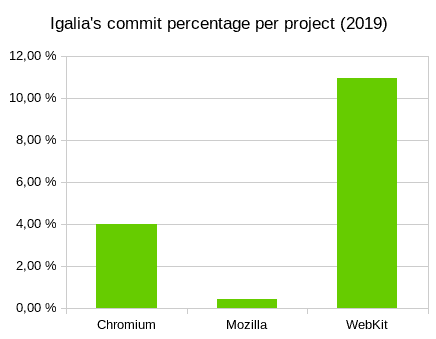Diagram showing, for each project, the percentage of Igalia's commits in 2019 relative to the total amount of the project. From left to right: Chromium (~3.96%), Mozilla (~0.43%) and WebKit (~10.92%).

In the WebKit project, where ~80% of the contributions were made by Apple, Igalia was second with ~10% of the total. In the Chromium project, the huge Google team made more than 90% of the contributions and many more companies are involved, but Igalia was second with about 4% of the total. In the Mozilla project, Mozilla is also doing ~90% of the contributions but Igalia only had ~0.5% of the total. Interestingly, the second contributing organization was… the community of unindentified gmail.com addresses! Of course, this shows the importance of volunteers in the Mozilla project where a great effort is done to encourage participation.

## Open Prioritization

From the commit count, it’s clear Igalia is not contributing as much to the Mozilla project as to Chromium or WebKit projects. But this is expected and is just reflecting the priority set by large companies. The solid base of Firefox users as well as the large amount of volunteer contributors show that the Mozilla project is nevertheless still attractive for many people. Could we turn this into browser development that is not funded by advertising or selling devices?

Another related question is whether the internet can really be shaped by the global community as defended by the Mozilla’s mission? Is the web doomed to be controlled by big corporations doing technology’s “evangelism” or lobbying at standardization committees? Are there prioritization issues that can be addressed by moving to a more collective decision process?

At Igalia, we internally try and follow a more democratic organization and, at our level, intend to make the world a better place. Today, we are launching a new Open Prioritization experiment to verify whether crowdfunding could be a way to influence how browser development is prioritized. Below is a short (5 min) introductory video:

I strongly recommend you to take a look at the proposed projects and read the FAQ to understand how this is going to work. But remember this is an experiment so we are starting with a few ideas that we selected and tasks that are relatively small. We know there are tons of user reports in bug trackers and suggestions of standards, but we are not going to solve everything in one day !

If the process is successful, we can consider generalizing this approach, but we need to test it first, check what works and what doesn’t, consider whether it is worth pursuing, analyze how it can be improved, etc

## Two Crowdfunding Tasks for Firefox

• CSS lab() colors. This is about giving web developers a way to express colors using the CIELAB color space which approximates better the human perception. My colleague Brian Kardell wrote a blog with more details. Some investigations have been made by Apple and Google. Let’s see what we can do for Firefox !
• SVG path d attribute. This is about expressing SVG path using the corresponding CSS syntax for example <path style="d: path('M0,0 L10,10,...')">. This will likely involve a refactoring to use the same parser for both SVG and CSS paths. It’s a small feature but part of a more general convergence effort between SVG and CSS that Igalia has been involved in.